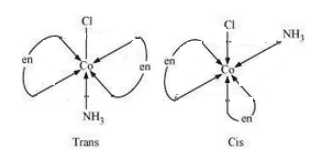# NCERT Solutions for Class 12 Chemistry Chapter 9 Coordination Compounds

NCERT solutions for class 12 chemistry chapter 9 Coordination Compounds - In the last chapter 8 The d and f block elements you have learnt that the transition metals form a large number of complex compounds. The complex compounds, the metal atoms are bound to a number of neutral molecules or anions by sharing electrons. Coordination compounds is a conceptual chapter related to chemical reactions and atoms so you must pay special attention to the NCERT solutions for class 12 chemistry chapter 9 Coordination Compounds which are provided here for free. Coordination compounds are an important part of inorganic chemistry and coordination compounds are the backbone of bio-inorganic and modern inorganic chemistry and the chemical industry. In this chapter, there are 11 intext questions and 32 questions in the exercise and you will find all the solutions of NCERT class 12 chapter 9 Coordination Compounds when you scroll down.

The NCERT solutions for class 12 chemistry chapter 9 Coordination Compounds are prepared and explained well by subject experts to help you in the preparation of CBSE Board exams. Also, the NCERT solutions for other classes and subjects can aid you in various competitive exams like JEE, NEET, BITS, and, KVPY etc. The seven sub-topics of chemistry chapter 9 Coordination Compounds covers important concepts like Werner’s Theory of coordination compounds, definitions of some important terms relating to coordination compounds, the rules of nomenclature of coordination compounds etc.

Intext Questions

Exercise Questions

After studying the chapter 9 Coordination Compounds of Class 12 Chemistry, you will be able to know the meaning of terms like, central atom or ion, coordination entity, ligand, coordination number, oxidation number, homoleptic and heteroleptic, able to learn the rules of nomenclature of coordination compounds, define various types of isomerism in coordination compounds and various applications of coordination compounds in daily life.

## Topics and Sub-topics of NCERT solutions for Class 12 Chemistry Chapter 9 Coordination Compounds-

9.1 Werner's Theory of Coordination Compounds

9.2 Definitions of Some Important Terms Pertaining to Coordination Compounds

9.3 Nomenclature of Coordination Compounds

9.4 Isomerism in Coordination Compounds

9.5 Bonding in Coordination Compounds

9.6 Bonding in Metal Carbonyls

9.7 Importance and Applications of Coordination Compounds

## Solutions to In-Text Questions Ex 9.1  to 9.10

(i) Tetraamminediaquacobalt(III) chloride

The chemical formula for the coordination compound Tetraamminediaquacobalt(III) chloride is

Question 9.1(ii) Write the formulas for the following coordination compounds:

(ii) Potassium tetracyanidonickelate(II)

The formula for the coordination compound Potassium tetracyanidonickelate II is :

Question 9.1(iii) Write the formulas for the following coordination compounds:

(iii) Tris(ethane–1,2–diamine) chromium(III) chloride

The formula for the coordination compound Tris(ethane–1,2–diamine) chromium(III) chloride is :

Question 9.1(iv) Write the formulas for the following coordination compounds:

(iv) Amminebromidochloridonitrito-N-platinate(II)

The formula for the coordination compound  Amminebromidochloridonitrito-N-platinate(II) :

(v) Dichloridobis(ethane–1,2–diamine)platinum(IV) nitrate

The chemical formula for the coordination compounds: Dichloridobis(ethane–1,2–diamine)platinum(IV) nitrate

Question 9.1(vi) Write the formulas for the following coordination compounds:

(vi) Iron(III) hexacyanidoferrate(II)

The formula for the coordination compound  Iron(III) hexacyanidoferrate(II):

The IUPAC name of the coordination compound     is :

Hexaamminecobalt(III) chloride

The IUPAC name of the coordination compound  is

Pentaamminechloridocobalt(III) chloride

Question 9.2(iii)  Write the IUPAC names of the following coordination compounds:

The IUPAC name of the coordination compound   is

Potassium hexacyanoferrate(III).

The IUPAC name of the following coordination compound     is

Potassium trioxalatoferrate(III)

The IUPAC names of the coordination compound    is

The IUPAC name of the following coordination compound   is :

Diamminechlorido(methylamine)platinum(II) chloride.

Both geometrical (cis-, trans-) and optical isomers exists for the given compound.Two optical isomers can exist, Dextro and Laevo.There are 10 possible isomers.There are geometrical, ionisation and linkage isomers possible.

A pair of optical isomerit also shows ionization isomerism

AND

It can also show linkage isomerism

AND

Geometrical (cis-, trans-) isomers can exists forQuestion 9.4(i)   Give evidence that following is ionisation isomer.

The ionisation isomers dissolve in water to yield different ions and thus react differently to various reagents:

[Co(NH3)5Br]SO4 + Ba2+ → BaSO4 (s)

[Co(NH3)5SO4]Br + Ba2+ → No reaction

[Co(NH3)5Br]SO4 + Ag+ → No reaction

[Co(NH3)5SO4]Br + Ag+ → AgBr (s)

Question 9.4(ii) Give evidence that following is ionisation isomer.

The ionisation isomers dissolve in water to yield different ions and thus react differently to various reagents:

[Co(NH3)5Br]SO4 + Ba2+ → BaSO4 (s)

[Co(NH3)5SO4]Br + Ba2+ → No reaction

[Co(NH3)5Br]SO4 + Ag+ → No reaction

[Co(NH3)5SO4]Br + Ag+ → AgBr (s)

In both the compounds, the oxidation state of Nickel is +2. So it has d8 configuration.

Now, on the basis of ligand pairing of electrons will occur. Since CN- is a strong ligand so pairing will occur but in case of  Cl- pairing will not be there as it is a weak ligand. So, the configuration of both the compounds looks like :-Thus   is a square planer and diamagnetic and    has tetrahedral geometry and is paramagnetic.

The difference in the magnetic behaviour is due to the nature of ligands present. In case of    the oxidation state of nickel is +2 and also Cl- is a weak ligand. Thus its configuration becomes:-So it is paramagnetic and tetrahedral in nature.

In the case of    ,  the oxidation state of nickel is 0. So its configuration is 3d8 4s2. We also know that CO is a strong ligand, thus the configuration of nickel becomes:-Hence the given compound is diamagnetic but tetrahedral in nature.

In both the compounds Fe has +3 oxidation state i.e., d5 configuration.In the case of strong ligand (CN-), the pairing of electron will occur. So number of electrons left unpaired will be 1.

In case of weak ligand (H2O), pairing of electron will not there. Thus number of electrons unpaired will be 5.

We know that paramagnetic strength is directly proportional to the number of unpaired electrons.

Hence paramagnetism will be more in case of

Firstly consider     :-

The oxidation state of cobalt is +3. So the electronic configuration of it will be d6.

Since (NH3) is a strong ligand so the pairing of electron will be there.So, it has d2sp3 hybridisation and an inner orbital complex.

Now consider,

The oxidation state of nickel is +3. So its electronic configuration will be d8.

Also (NH3) is a strong ligand so pairing of electrons will be seen.Thus      is an outer orbital complex.

The oxidation state of Pt in the given compound is +2. Also, it is given that the compound has square planar geometry i.e., it has dsp2 hybridisation (d8).

CN is a strong ligand so the pairing of electron will occur.

So there are be no unpaired electrons in the given compound.

Consider Hexaqua manganese (II) :- In this compound, the oxidation state of Mn is +2 and its electronic configuration is d5.

H2O is a weak ligand and crystal field is octahedral so the arrangement of electrons will be   t23g eg2.

So the total number of unpaired electrons is 5.

Now consider hexacyanoion:-  In this compound, the oxidation state of Mn is +2. It is surrounded by the strong ligands CN-, so pairing will be there.

Its arrangement will be   t2g5 eg0.

Thus the number of unpaired electrons will be 1.

## NCERT Solutions for Class 12 Chemistry Chapter 9 Coordination Compounds- Exercise Questions

Werner his theory of coordination compounds and gave some postulates. The main postulates are:

1. In coordination compounds metals show two types of linkages or valences namely primary valency and secondary valency.

2. The primary valences are generally ionisable and are satisfied or balanced by negative ions.

3. The secondary valences are non-ionisable. These are satisfied by either neutral molecules or by negative ions. The secondary valence is equal to the coordination number (No. of atoms surrounding the metal) and is constant for a metal.

4. According to different coordination numbers, the ions/groups bound by the secondary linkages to the metal have characteristic spatial arrangements.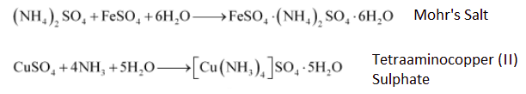The major difference between both the compounds is that the first compound is a salt and the other one is a coordination compound. In case of double salt compounds (Mohr's salt), the compound breaks into its constituent ions when dissolved in water, therefore it gives a positive test for the presence of Fe+2. But in case of coordination compounds, they maintain their identity in both solid and dissolved state. Thus the individual property of each constituent is lost. And therefore it doesn't give a positive test for Cu+2.

(i) Coordination entity:-   It is an electrically charged species carrying either a positive charge or a negative charge. In a coordination entity, the central atom or ion is surrounded by some number of neutral molecules or negative ions ( called ligands) accordingly.

(ii) Ligand:- Ligands are the neutral molecules or negatively charged ions that surround the metal atom in a coordination entity according to the holding capacity of central metal ion are known as ligands. NH3  and  H2O are two neutral ligands.

(iii) Coordination number:-  The total number of metals that surrounds the central metal ion is known as coordination number.

For e.g,(a) In case of six chlorine atoms are attached to Pt, thus the coordination number of the given compound is 6.

(b) In case of  the central metal ion Ni is surrounded by 4 atoms of ligand so its coordination number is 4.

(iv)  Coordination polyhedron:-  It is defined as the spatial arrangement of the ligands which are directly attached to the central metal ion/atom.

E.g.  square planar,  tetrahedral.

(v) Homoleptic:-  Homoleptic compounds are defined as the compounds in which the donor/ligand attached to central metal atom/ion is of one kind.

E.g.     and

(vi) Heteroleptic:-  These are the coordination compounds in which central atoms are attached with more than one type of ligand.

E.g.         and

Unidentate ligands:-   The ligand which has only one donor site are known as unidentate ligands. E.g.  Cl -, NH3

Bidentate(or didentate):-The ligands which have two donor sites are known as bidentate ligands.  E.g.  Ethane - 1,2 diamine, Oxalate ion

Ambidentate ligands:-  The ligands which can attach themselves to the central metal through two different atoms. E.g  NO2, SCN

Let us assume that coordination number of cobalt is x.

Thus

Hence coordination number of cobalt is +3.

Let us assume that the coordination number of cobalt is x.

Then according to the question :

Hence the coordination number of cobalt is 3.

Let us assume the oxidation state of Pt to be x.

Then the equation becomes :-

Hence the oxidation state of Pt metal is 2.

Let the oxidation state of Fe be x.

Then the equation will be :-

Thus oxidation state of Fe in this coordination compound is 3.

Let us assume the oxidation state of Cr to be x.

Then according to the question we get,

So the oxidation state of Cr will be 3.

Question 9.6(i)     Using IUPAC norms write the formulas for the following:

(i) Tetrahydroxidozincate(II)

Using the IUPAC rules :-

Question 9.6(ii)     Using IUPAC norms write the formulas for the following:

The required compound is :-

Question 9.6(iii)     Using IUPAC norms write the formulas for the following:

(iii) Diamminedichloridoplatinum(II)

The required chemical formula of compound is :-

Question 9.6(viii)     Using IUPAC norms write the formulas for the following:

(viii) Hexaammineplatinum(IV)

The required compound is :-

Using IUPAC norms the name of the given compound is :-

Hexaamminecobalt(III) chloride

According to the IUPAC norms :-

Diamminechlorido(methylamine) platinum(II) chloride

Question 9.7(iii)     Using IUPAC norms write the systematic names of the following:

Using the nomenclature rules, the required name of the compound is:-   Hexaquatitanium(III) ion

Using IUPAC norms, the name of the given compound is :-

Tetraamminichloridonitrito-N-Cobalt(III) chloride

The IUPAC name of the givne compound is :-

Hexaquamanganese(II) ion

Question9.7(vi)     Using IUPAC norms write the systematic names of the following:

According to the IUPAC norms, the systematic name of the given compound is:-     Tetrachloridonickelate(II) ion

Question 9.7(vii)     Using IUPAC norms write the systematic names of the following:

The IUPAC name of the given compound is :-   Hexaamminenickel(II) chloride

Question 9.7(viii)     Using IUPAC norms write the systematic names of the following:

The name of the given compound is :-      Tris(ethane-1, 2-diammine) cobalt(III) ion

The IUPAC name of the given compound is :-    Tetracarbonylnickel(0)

Two main types of isomerism are known in case of coordination compounds which can be further divided into subgroups:-

(a) Stereoisomerism   :-

(i)  Geometrical isomerism   E.g

.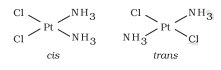(ii)  Optical isomerism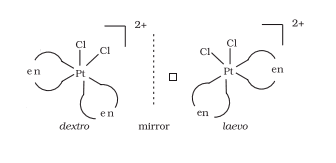(b)  Structural isomerism :-

(ii) Ionisation isomerism        E.g      and

(iii) Coordination isomerism   E.g        and

(iv) Solvate isomerism           E.g           and

No geometrical isomers are possible since the given compound is a bidentate compound.

The facial (fac) and meridional (mer) isomers are possible for the given compound.Question 9.10(i)     Draw the structures of optical isomers of:

The optical isomers of the given compound are:-Question 9.10(ii)     Draw the structures of optical isomers of:

The optical isomers of the given compound are given below  :-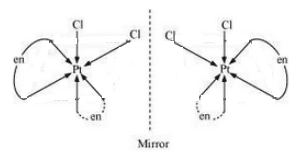Question 9.10(iii)     Draw the structures of optical isomers of:

The optical isomers of the given compound are:-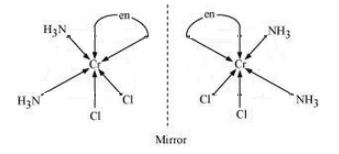Question 9.11(i)     Draw all the isomers (geometrical and optical) of:

The configurational isomers are:-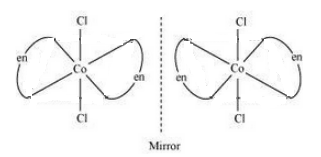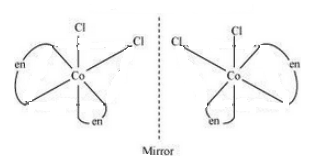Question 9.11(ii)     Draw all the isomers (geometrical and optical) of: